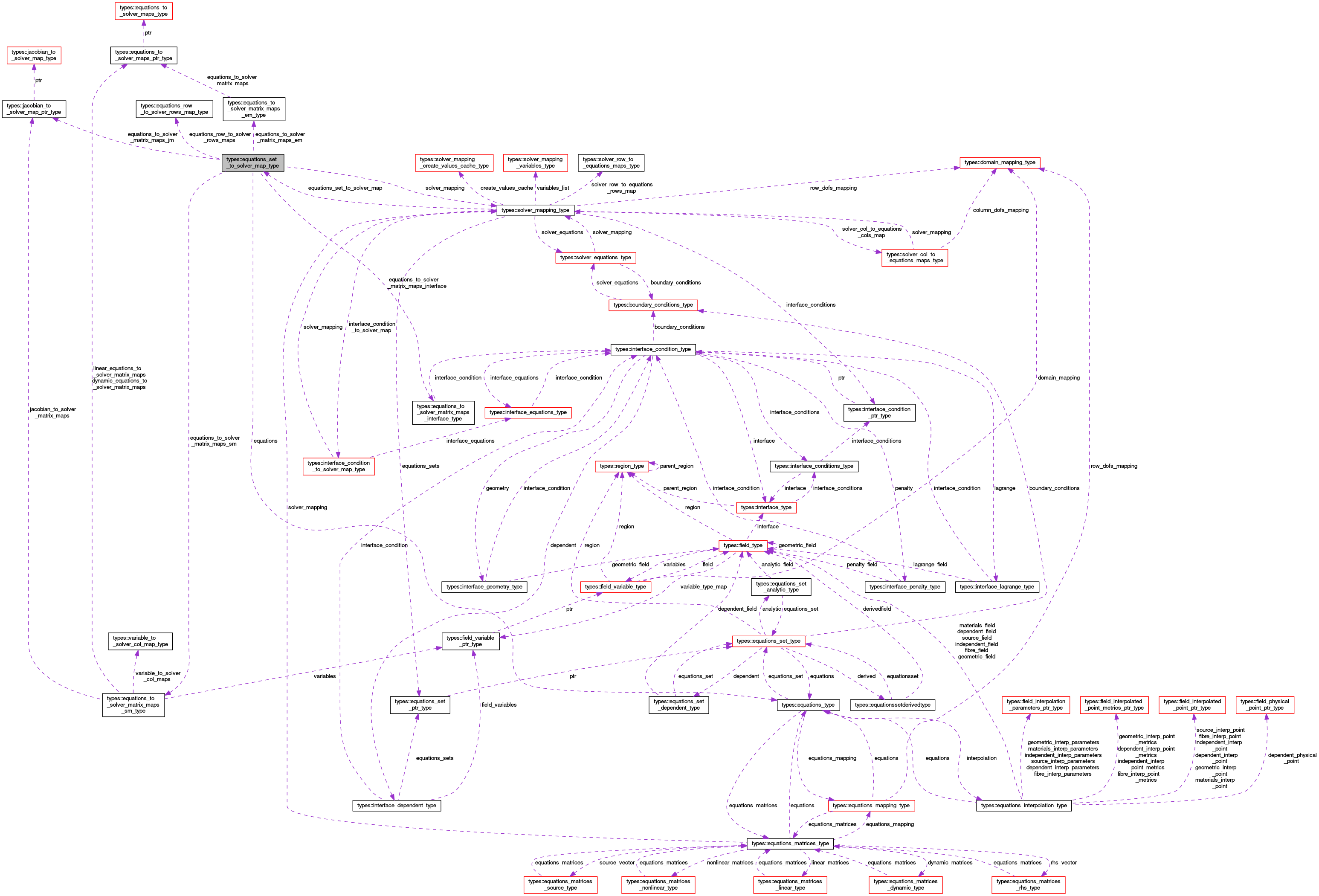OpenCMISS-Iron Internal API Documentation
types::equations_set_to_solver_map_type Type Reference

Contains information on the mappings from an equations set to the solver matrices. More...

Collaboration diagram for types::equations_set_to_solver_map_type:[legend]

## Public Attributes

integer(intg) equations_set_index
The index of the equations set for these mappings. More...

type(solver_mapping_type), pointer solver_mapping
A pointer to the solver mappings. More...

type(equations_type), pointer equations
A pointer to the equations in this equations set. More...

integer(intg) number_of_interface_conditions
The number of interface conditions affecting this equations set. More...

type(equations_to_solver_matrix_maps_interface_type), dimension(:), allocatable equations_to_solver_matrix_maps_interface
EQUATIONS_TO_SOLVER_MATRIX_MAPS_INTERFACE(interface_condition_idx). Information on the interface_condition_idx'th interface condition affecting this equations set. More...

type(equations_to_solver_matrix_maps_sm_type), dimension(:), allocatable equations_to_solver_matrix_maps_sm
EQUATIONS_TO_SOLVER_MATRIX_MAPS_SM(solver_matrix_idx). The mappings from the equations matrices in this equation set to the solver_matrix_idx'th solver_matrix. More...

type(equations_to_solver_matrix_maps_em_type), dimension(:), allocatable equations_to_solver_matrix_maps_em
EQUATIONS_TO_SOLVER_MATRIX_MAPS_EM(equations_matrix_idx). The mappings from the equation_matrix_idx'th equations matrix in this equation set to the solver_matrices. More...

type(jacobian_to_solver_map_ptr_type), dimension(:), allocatable equations_to_solver_matrix_maps_jm
EQUATIONS_TO_SOLVER_MATRIX_MAPS_JM(jacobian_idx). The mappings from the jacobian_idx'th Jacobian matrix in this equation set to the solver_matrices. More...

type(equations_row_to_solver_rows_map_type), dimension(:), allocatable equations_row_to_solver_rows_maps
EQUATIONS_ROW_TO_SOLVER_ROWS_MAPS(equations_row_idx). The mappings from the equations_row_idx'th equations row to the solver matrices rows. More...

## Detailed Description

Contains information on the mappings from an equations set to the solver matrices.

Definition at line 2912 of file types.f90.

## Member Data Documentation

 type(equations_type), pointer types::equations_set_to_solver_map_type::equations

A pointer to the equations in this equations set.

Definition at line 2915 of file types.f90.

 type(equations_row_to_solver_rows_map_type), dimension(:), allocatable types::equations_set_to_solver_map_type::equations_row_to_solver_rows_maps

EQUATIONS_ROW_TO_SOLVER_ROWS_MAPS(equations_row_idx). The mappings from the equations_row_idx'th equations row to the solver matrices rows.

Definition at line 2921 of file types.f90.

 integer(intg) types::equations_set_to_solver_map_type::equations_set_index

The index of the equations set for these mappings.

Definition at line 2913 of file types.f90.

 type(equations_to_solver_matrix_maps_em_type), dimension(:), allocatable types::equations_set_to_solver_map_type::equations_to_solver_matrix_maps_em

EQUATIONS_TO_SOLVER_MATRIX_MAPS_EM(equations_matrix_idx). The mappings from the equation_matrix_idx'th equations matrix in this equation set to the solver_matrices.

Definition at line 2919 of file types.f90.

 type(equations_to_solver_matrix_maps_interface_type), dimension(:), allocatable types::equations_set_to_solver_map_type::equations_to_solver_matrix_maps_interface

EQUATIONS_TO_SOLVER_MATRIX_MAPS_INTERFACE(interface_condition_idx). Information on the interface_condition_idx'th interface condition affecting this equations set.

Definition at line 2917 of file types.f90.

 type(jacobian_to_solver_map_ptr_type), dimension(:), allocatable types::equations_set_to_solver_map_type::equations_to_solver_matrix_maps_jm

EQUATIONS_TO_SOLVER_MATRIX_MAPS_JM(jacobian_idx). The mappings from the jacobian_idx'th Jacobian matrix in this equation set to the solver_matrices.

Definition at line 2920 of file types.f90.

 type(equations_to_solver_matrix_maps_sm_type), dimension(:), allocatable types::equations_set_to_solver_map_type::equations_to_solver_matrix_maps_sm

EQUATIONS_TO_SOLVER_MATRIX_MAPS_SM(solver_matrix_idx). The mappings from the equations matrices in this equation set to the solver_matrix_idx'th solver_matrix.

Definition at line 2918 of file types.f90.

 integer(intg) types::equations_set_to_solver_map_type::number_of_interface_conditions

The number of interface conditions affecting this equations set.

Definition at line 2916 of file types.f90.

 type(solver_mapping_type), pointer types::equations_set_to_solver_map_type::solver_mapping

A pointer to the solver mappings.

Definition at line 2914 of file types.f90.